# Steps

Peter makes steps long 70 cm, John 45 cm long. After how many meters their footsteps meet?

x =  6.3 m

### Step-by-step explanation:Did you find an error or inaccuracy? Feel free to write us. Thank you!Tips to related online calculators
Do you want to calculate least common multiple two or more numbers?
Do you want to convert length units?

## Related math problems and questions:

• The classroomThe classroom is 9 meters long. The width of the classroom is smaller and can be passed in equally long steps of 55 CM or 70 CM. Determine the width of the classroom.
• Lcm simpleFind least common multiple of this two numbers: 140 175.
• Steps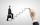An adult step has a length 76 cm. How many steps will he go distance 50 meters? How many meters does he go when he makes 700 steps?
• Square roomWhat is the size of the smallest square room, which can pave with tiles with dimensions 55 cm and 45 cm? How many such tiles is needed?
• LCMWhat is the least common multiple of 5, 50, 14?
• The King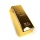The King wants to divide his sons equally. He has 42 rubies and 45 diamonds. How many sons and how will they share them?
• Square tilesThe room has dimensions of 12 meters and 5.6 meters. Determine the number of square tiles and their largest dimension to exactly cover the floor.
• Trams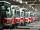Tram no. 3,7,10,11 rode together from the depot at 5am. Tram No. 3 returns after 2 hours, tram No. 7 an hour and half, no. 10 in 45 minutes and no. 11 in 30 minutes. For how many minutes and when these trams meet again?
• CubesCarol with cut bar 12 cm x 12 cm x 135 cm to the cubes. Find the sum of all the surfaces of the resulting cubes.
• PexesoDrawing paper has dimensions of 60cm and 840mm. Pupils have to divide it into squares so that they can make a pexeso. What dimension must squares have if their side is to be larger than 3cm and less than 10cm?
• Bathtub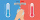Kevin has a bathtub shaped like a block. The bathtub measures 70 cm long, 45 cm wide and 50 cm high. Then the bathtub will be filled with clean water 3/5 of its height. The clean water needed is….
• Glass panelA rectangular glass panel with dimensions of 72 cm and 96 cm will cut the glazier on the largest square possible. What is the length of the side of each square? How many squares does the glazier cut?
• FlowerbedsWhat is the smallest length of flowerbeds we have to prepare to plant seedlings at grid 20,30,25,40 cm?
• Lcm of three numbersWhat is the Lcm of 120 15 and 5
• Tram stop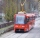The blue tram stops every 12 minutes, the red one 8 minutes. At 8 o'clock they left the stop together. How many times do they meet at a stop before 11 am?1st blade 2,5 m, 2nd blade. .1.75 m. How many same long pieces of this two blades can be do the biggest? How long is one piece?Find the smallest multiple of 63 and 147